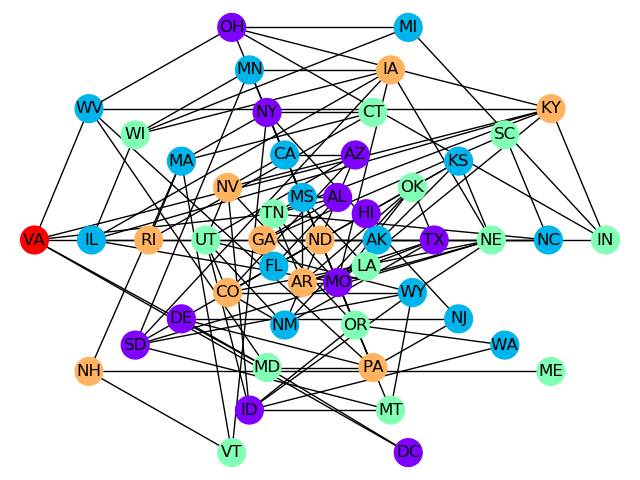# Large Map Coloring¶

This example solves a map coloring problem to demonstrate an out-of-the-box use of Ocean’s classical-quantum hybrid sampler, dwave-hybrid Kerberos, that enables you to solve problems of arbitrary structure and size.

Map coloring is an example of a constraint satisfaction problem (CSP). CSPs require that all a problem’s variables be assigned values, out of a finite domain, that result in the satisfying of all constraints. The map-coloring CSP is to assign a color to each region of a map such that any two regions sharing a border have different colors.

The Map Coloring advanced example demonstrates lower-level coding of a similar problem, which gives the user more control over the solution procedure but requires the knowledge of some system parameters (e.g., knowing the maximum number of supported variables for the problem). Example Problem With Many Variables demonstrates the hybrid approach to problem solving in more detail by explicitly configuring the classical and quantum workflows.

## Example Requirements¶

To run the code in this example, the following is required.

If you installed dwave-ocean-sdk and ran dwave config create, your installation should meet these requirements.

## Solution Steps¶

Section Solving Problems on a D-Wave System describes the process of solving problems on the quantum computer in two steps: (1) Formulate the problem as a binary quadratic model (BQM) and (2) Solve the BQM with a D-wave system or classical sampler. In this example, a function in Ocean software handles both steps. Our task is mainly to select the sampler used to solve the problem.

## Formulate the Problem¶

This example uses the NetworkX read_adjlist function to read a text file, usa.adj, containing the states of the USA and their adjacencies (states with a shared border) into a graph. The original map information was found here on write-only blog of Gregg Lind and looks like this:

# Author Gregg Lind
# License:  Public Domain.    I would love to hear about any projects you use if it for though!
#
AK,HI
AL,MS,TN,GA,FL
AR,MO,TN,MS,LA,TX,OK
AZ,CA,NV,UT,CO,NM
CA,OR,NV,AZ
CO,WY,NE,KS,OK,NM,AZ,UT

# Snipped here for brevity


You can see in the first non-comment line that the state of Alaska (“AK”) has Hawaii (“HI”) as an adjacency and that Alabama (“AL”) shares borders with four states.

>>> import networkx as nx


Graph G now represents states as vertices and each state’s neighbors as shared edges. Ocean’s dwave_networkx can return a minimum vertex coloring for a graph, which assigns a color to the vertices of a graph in a way that no adjacent vertices have the same color, using the minimum number of colors. Given a graph representing a map and a sampler, the min_vertex_coloring function tries to solve the map coloring problem.

dwave-hybrid Kerberos is classical-quantum hybrid asynchronous decomposition sampler, which can decompose large problems into smaller pieces that it can run both classically (on your local machine) and on the D-Wave system. Kerberos finds best samples by running in parallel tabu search, simulated annealing, and D-Wave subproblem sampling on problem variables that have high impact. The only optional parameters set here are a maximum number of iterations and number of iterations with no improvement that terminates sampling. (See the Problem With Many Variables example for more details on configuring the classical and quantum workflows.)

>>> import dwave_networkx as dnx
>>> from hybrid.reference.kerberos import KerberosSampler
>>> coloring = dnx.min_vertex_coloring(G, sampler=KerberosSampler(), chromatic_ub=4, max_iter=10, convergence=3)
>>> set(coloring.values())
{0, 1, 2, 3}


Note

The next code requires Matplotlib.

Plot the solution, if valid.

>>> import matplotlib.pyplot as plt
>>> node_colors = [coloring.get(node) for node in G.nodes()]
>>> if dnx.is_vertex_coloring(G, coloring):  # adjust the next line if using a different map
...    nx.draw(G, pos=nx.shell_layout(G, nlist = [list(G.nodes)[x:x+10] for x in range(0, 50, 10)] + [[list(G.nodes)]]), with_labels=True, node_color=node_colors, node_size=400, cmap=plt.cm.rainbow)
>>> plt.show()


The graphic below shows the result of one such run.One solution found for the USA map-coloring problem.